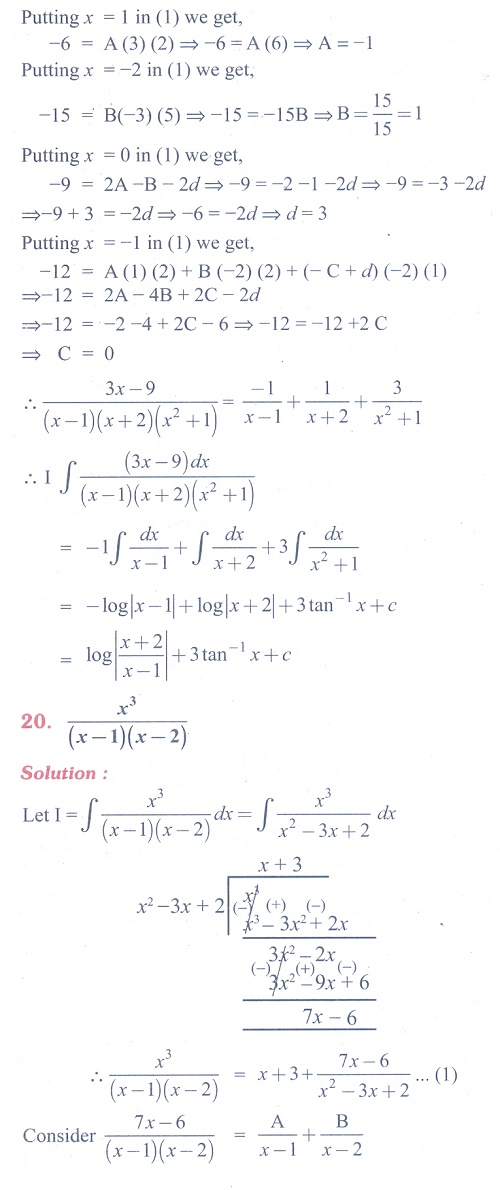Home | | Maths 11th std | Exercise 11.5: Decomposition by Partial Fractions - Integration Method

# Exercise 11.5: Decomposition by Partial Fractions - Integration Method

Maths Book back answers and solution for Exercise questions - Mathematics : Integral Calculus: Decomposition by Partial Fractions - Integration MethodTags : Problem Questions with Answer, Solution | Mathematics , 11th Mathematics : UNIT 11 : Integral Calculus
Study Material, Lecturing Notes, Assignment, Reference, Wiki description explanation, brief detail
11th Mathematics : UNIT 11 : Integral Calculus : Exercise 11.5: Decomposition by Partial Fractions - Integration Method | Problem Questions with Answer, Solution | Mathematics